Question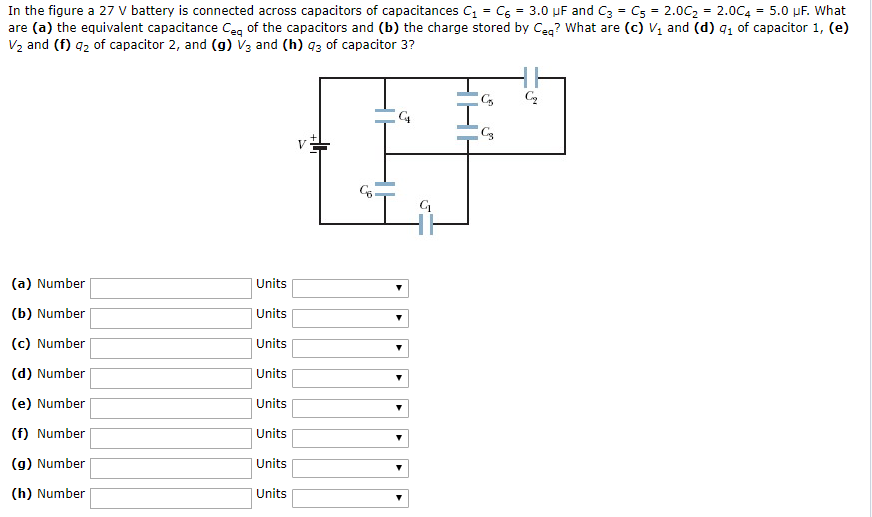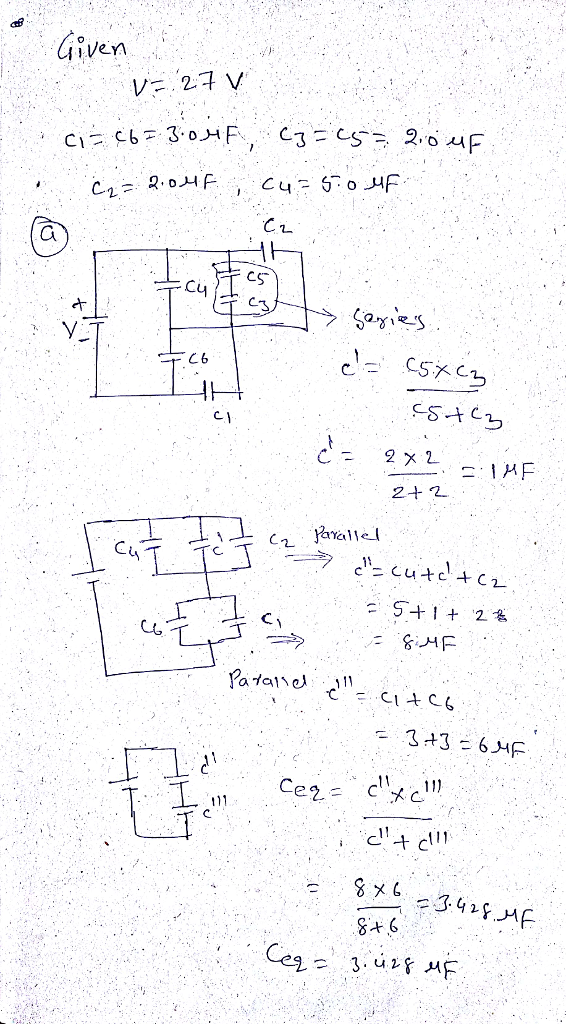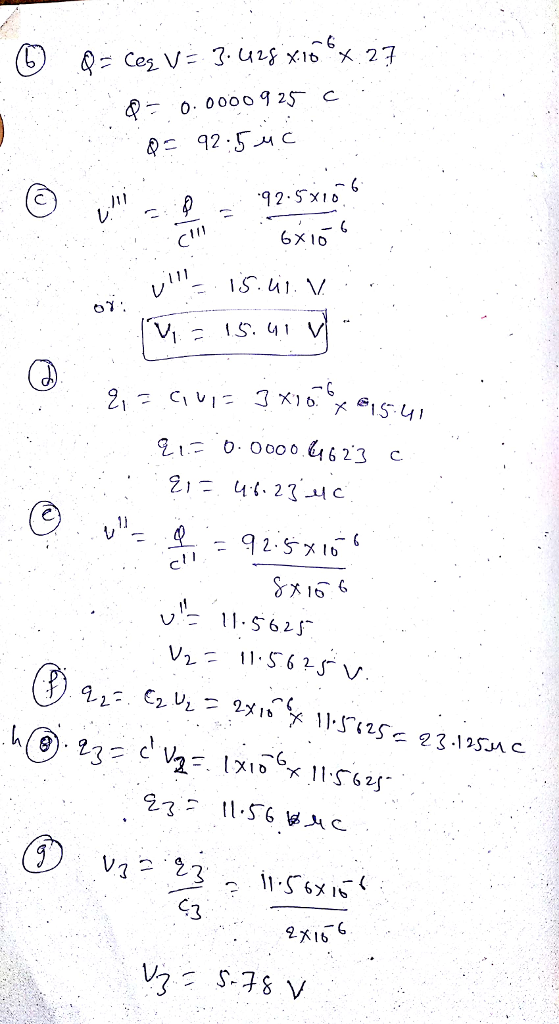#### Earn Coins

Coins can be redeemed for fabulous gifts.

Similar Homework Help Questions
• ### In the figure a 27 V battery is connected across capacitors of capacitances C1 = C6...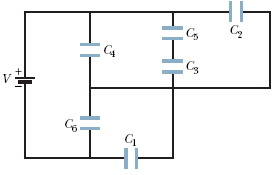In the figure a 27 V battery is connected across capacitors of capacitances C1 = C6 = 5.0 μF and C3 = C5 = 1.5C2 = 1.5C4 = 6.0 μF. What are (a) the equivalent capacitance Ceq of the capacitors and (b) the charge stored by Ceq? What are (c) V1 and (d) q1 of capacitor 1, (e) V2 and (f) q2 of capacitor 2, and (g) V3 and (h) q3 of capacitor 3? C HHH

• ### In the figure a 23 V battery is connected across capacitors of capacitances C1 = C6 = 5.0 μF and C3 = C5=...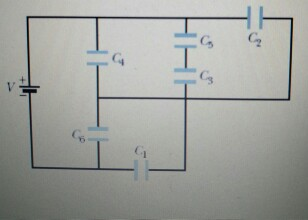In the figure a 23 V battery is connected across capacitors of capacitances C1 = C6 = 5.0 μF and C3 = C5= 2.0C2 = 2.0C4 = 5.0 μF. What are (a) the equivalent capacitance Ceq of the capacitors and (b) the charge stored by Ceq? What are (c) V1 and (d) q1 of capacitor 1,(e) V2 and (f) q2 of capacitor 2, and (g) V3 and (h) q3 of capacitor 3?

• ### In the figure a 27 V battery is connected across capacitors of capacitances C1-C6 5.0 μF...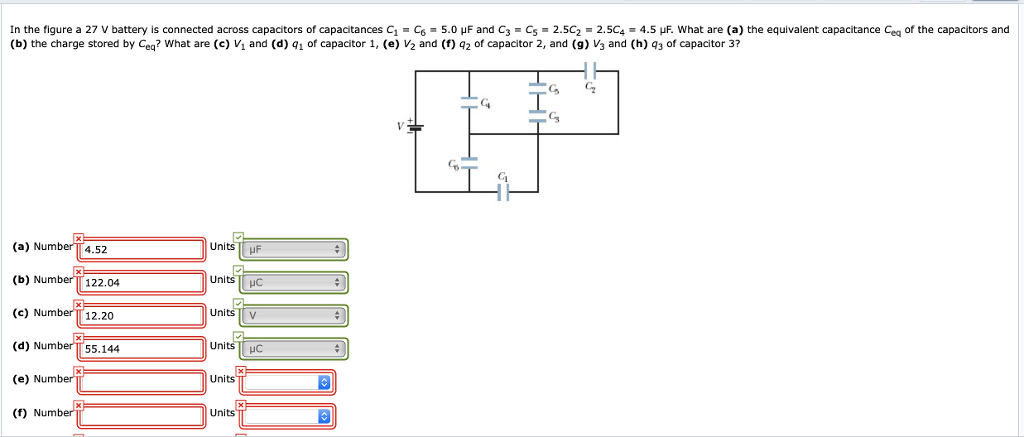In the figure a 27 V battery is connected across capacitors of capacitances C1-C6 5.0 μF and C3-C5-2.5C2-2.5C4-4.5 what are (a) the equivalent capacitance Ceq of the capacitors and (b) the charge stored by Ceq? What are (c) V and (d) q1 of capacitor 1, (e) V2 and (f) q2 of capacitor 2, and (g) V3 and (h) 43 of capacitor 3? G C Ci Gi (a) Number (b) Number 122.04 (c) Number 12.20 (d) Number 55.144 (e) Number (f)...

• ### In the figure a 15 V battery is connected across capacitors of capacitances C1-Cg = 3.0...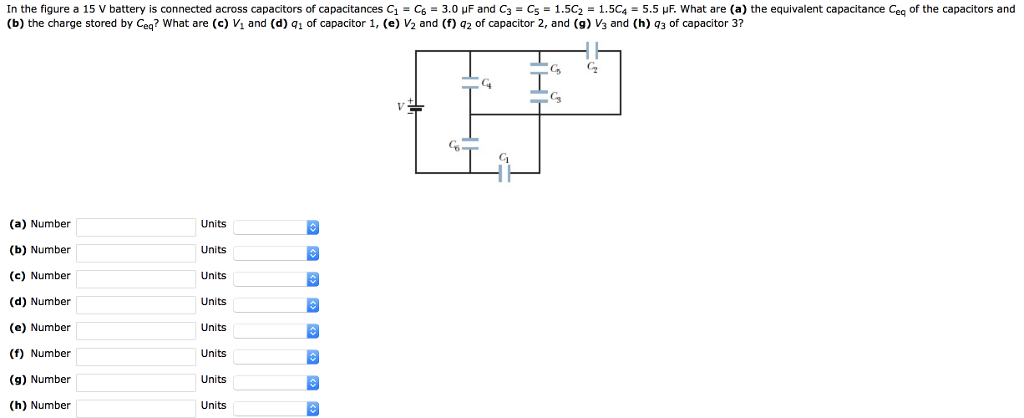In the figure a 15 V battery is connected across capacitors of capacitances C1-Cg = 3.0 μF and C3-C5-1.5C2-1.5C4-5.5岠what are (a) the equivalent capacitance Ceq of the capacitors and (b) the charge stored by Ceq? What are (c) Vi and (d) qi of capacitor 1, (e) V2 and (f) q2 of capacitor 2, and (9) V3 and (h) q3 of capacitor 3? G C C3 G6 (a) Number (b) Number (c) Number (d) Number (e) Number (f) Number (g) Number...

• ### In the figure a 15 V battery is connected across capacitors of capacitances C1-Cg = 3.0...In the figure a 15 V battery is connected across capacitors of capacitances C1-Cg = 3.0 μF and C3-C5-1.5C2-1.5C4-5.5岠what are (a) the equivalent capacitance Ceq of the capacitors and (b) the charge stored by Ceq? What are (c) Vi and (d) qi of capacitor 1, (e) V2 and (f) q2 of capacitor 2, and (9) V3 and (h) q3 of capacitor 3? G C C3 G6 (a) Number (b) Number (c) Number (d) Number (e) Number (f) Number (g) Number...

• ### Potential across a CapacitorIn Fig. 25-31 a20.0 V battery is connected across capacitors of capacitances C1 = C6 = 2.9 μF and C3 = C5 = 2.00C2 = 2.00C4 = 4.7 μF.Fig. 25-31http://i.imgur.com/IudoK.jpgWhat are(a) the equivalent capacitance Ceq of the capacitorsCeq = 3.18(b) the charge stored by Ceq?q = 63.6What are(c) V1 and (d) q1 of capacitor 1,V1 =q1 = (e) V2(f) q2 of capacitor 2,V2 = V q2 = (g) V3 and (h) q3 of capacitor 3,V3 =q3 =

• ### in the ngure a s v battery is connected across capacitors of capacitances c.-cs = 4.0...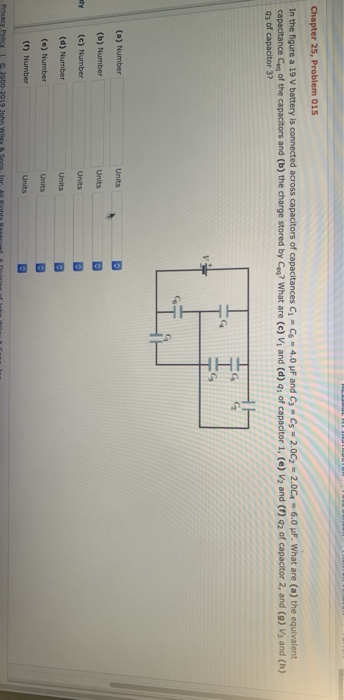in the ngure a s v battery is connected across capacitors of capacitances c.-cs = 4.0 μF and C3-C5 = 2.0C2-2004-6.0 μF. What are (a) the equivalent capacitance of the capacitors and (b) the charge stored by ㎝? what are (c) vi and (d) q1 of capacitor 1, (e) V2 and (f) q2 of capacitor 2, and (g) Vg and (h) 43 of capacitor 3? (a) Number (b) Number dy (c) Number (d) Number (e) Number (1) Number (a) Number...

• ### Q22 (Capacitors) The figure below shows a 15.0 V battery and four uncharged capacitors of capacitances...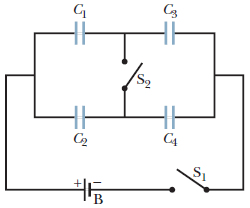Q22 (Capacitors) The figure below shows a 15.0 V battery and four uncharged capacitors of capacitances C1 = 8.00 μF, C2 = 4.00 μF, C3 = 6.00 μF, and C4 = 3.00 μF. If only switch S1 is closed, what is the charge on each capacitor? (a)    C1 __________ μC (b)    C2 __________ μC (c)    C3 __________μC (d)    C4 __________ μC If both switches are closed, what is the charge on each capacitor? (e)    C1 __________μC (f )    C2 __________μC (g)    C3 __________ μC (h)    C4 __________ μC...

• ### The figure shows a 9.18 V battery and four uncharged capacitors of capacitances C1 = 1.21...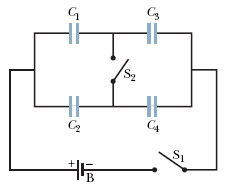The figure shows a 9.18 V battery and four uncharged capacitors of capacitances C1 = 1.21 ?F,C2 = 2.20 ?F,C3 = 3.21 ?F, and C4 = 4.25 ?F. If only switch S1 is closed, what is the charge on (a) capacitor 1, (b) capacitor 2, (c) capacitor 3, and (d) capacitor 4? If both switches are closed, what is the charge on (e) capacitor 1, (f) capacitor 2, (g) capacitor 3, and (h) capacitor 4? Cs So C2 C4

• ### The figure displays a 12.1 V battery and three uncharged capacitors of capacitances C1 = 4.11...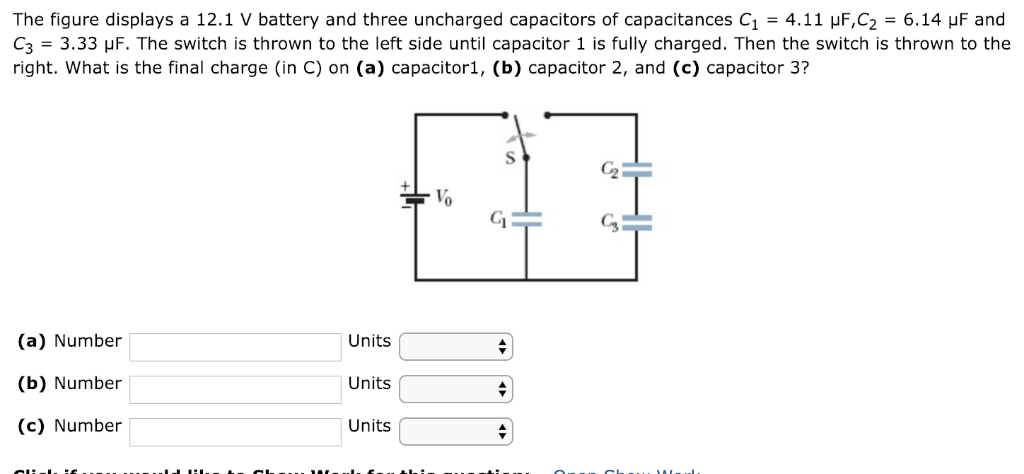The figure displays a 12.1 V battery and three uncharged capacitors of capacitances C1 = 4.11 μF,C2 = 6.14 μF and C3 = 3.33 μF. The switch is thrown to the left side until capacitor 1 is fully charged. Then the switch is thrown to the right. What is the final charge (in C) on (a) capacitor1, (b) capacitor 2, and (c) capacitor 3? Cs (a) Number (b) Number (c) Number Units Units Units